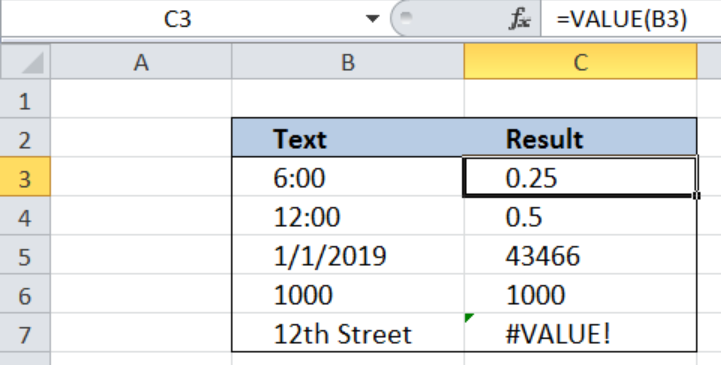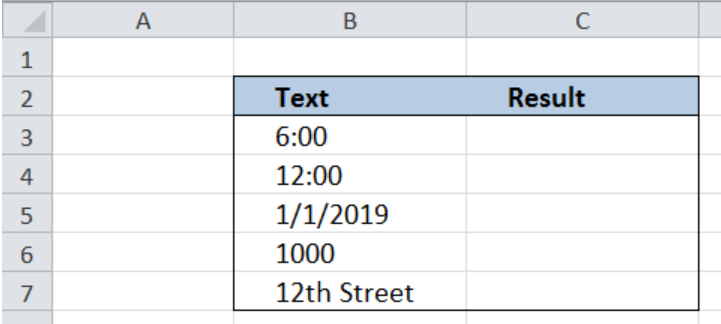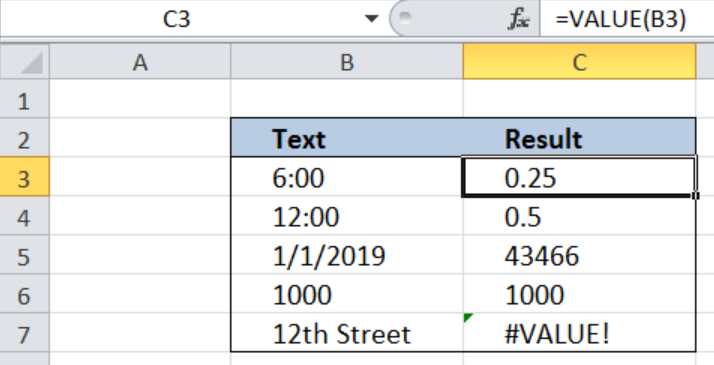Get instant live expert help with Excel or Google Sheets“My Excelchat expert helped me in less than 20 minutes, saving me what would have been 5 hours of work!”

#### Post your problem and you'll get expert help in seconds

Your message must be at least 40 characters
Our professional experts are available now. Your privacy is guaranteed.

# Excel VALUE Function

While working with Excel, we are able to convert any text into a numeric value by using the VALUE function.  This step by step tutorial will assist all levels of Excel users in the usage and syntax of the VALUE function.Figure 1. Final result:  Excel VALUE function

## Syntax of the VALUE Function

`=VALUE(text)`

• text – a text that we want to convert to a numeric value; text can either be enclosed in quotation marks or a cell reference
• text can be in time or date format, or a constant number; if text is in a format other than these three, VALUE function returns the error value “#VALUE!

## Setting up the Data

Our table consists of two columns: Text (column B) and Result (column C).  Column B contains a list of texts we want to convert into numeric values. The results will be recorded in column C.Figure 2. Sample data to convert to numeric values

## Convert text into numeric value

In order to convert the text in column B into numeric values, we will follow these steps:

Step 1.  Select cell C3

Step 2.  Enter the formula:`=``VALUE(B3)`

Step 3.  Press Enter

Step 4:  Copy the formula in cell C3 to cells C4:C7 by clicking the “+” icon at the bottom-right corner of cell C3 and dragging it downFigure 3. Entering the formula to convert to numeric values

The result in cell C3 is the numeric value of “6:00” which is 0.25.  Excel has a unique way of converting time into numbers, relative to a 24-hour period in one day.  Cells C4 to C7 show the corresponding numeric values of the text in B4:B6, whether the text is a constant number, in date format or in time format.

In cell C7, our formula returns a #VALUE! Error, because the text we want to convert, “12th Street”,  has no equivalent numeric value.

The VALUE function is generally not used because Excel normally converts text into a numeric value without using any function.  This function is available in Excel for compatibility with other spreadsheet programs.

Most of the time, the problem you will need to solve will be more complex than a simple application of a formula or function. If you want to save hours of research and frustration, try our live Excelchat service! Our Excel Experts are available 24/7 to answer any Excel question you may have. We guarantee a connection within 30 seconds and a customized solution within 20 minutes.

### Did this post not answer your question? Get a solution from connecting with the expert.Another blog reader asked this question today on Excelchat:
Related blogs## Subscribe to Excelchat.coAnother blog reader asked this question today on Excelchat: